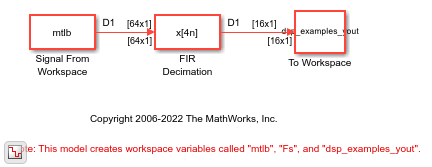# FIR Decimation Using Single-Rate Processing

In this example, the FIR Decimation block decimates the signal by decreasing the frame size, while keeping the same sample rate.

Open the model. The input to the FIR Decimation block is a single-channel input with a frame size of 64. In the block dialog box, set the Decimation factor parameter to 4 and the Rate options parameter to `Enforce single-rate processing`.

Run the Model. The block operates in the single-rate processing mode. Therefore, the output frame size of the block istimes the input frame size of the block, where M is the decimation factor. With a decimation factor of 4, the output has a frame size of 16. As shown in the model, the input and the output of the FIR Decimation block have the same sample rate and different frame sizes.## SupportGet trial now